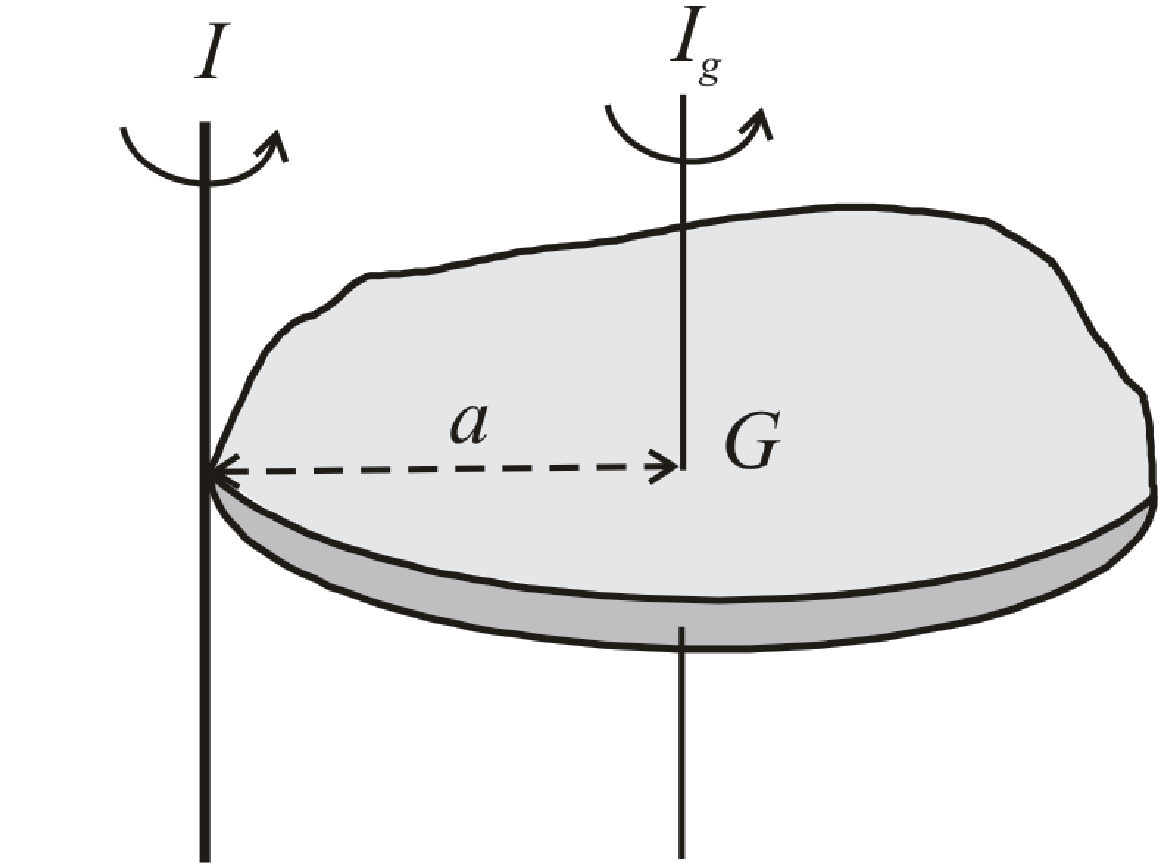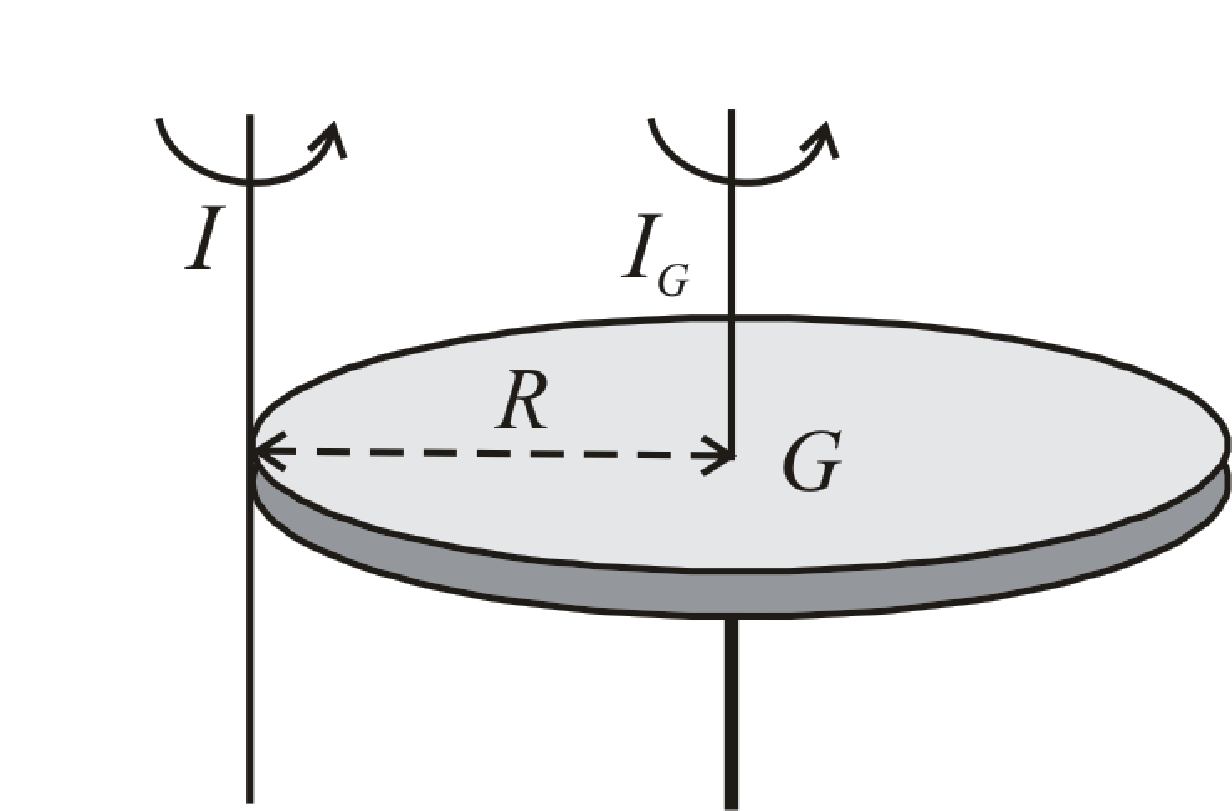BodhiAI
Oct. 31, 2019

#### Parallel Axis Theorem:

Theorem of parallel axes :

Moment of inertia of a body about a given axis I is equal to the sum of moment of inertia of the body about an axis parallel to given axis and passing through centre of mass of the body Ig and Ma2 where M is the mass of the body and a is the perpendicular distance between the two axes.I = Ig + Ma2

Example : Moment of inertia of a disc about an axis through its centre and perpendicular to the plane is 1/2MR2 so moment of inertia of the disc about an axis through its tangent and perpendicular to the plane will beI = Ig + Ma2